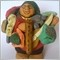• Get access

# Trailing Stop for a grid: is it feasible?95

Hi All,

I am trying to setup a trailing stop for a grid which includes a series of orders (long and short) for the same cross.

At the moment I can manage for the grid to close when a certain profit % has been hit, however what I would like to do is to create a sort of moving profit target loosely based on the logic of the trailing stop.

The challenge that I have here is that the Stop Loss of the entire grid should move up only if at the next tick the profit level increases otherwise it should stay the same. So I would need a way to store the max profit level reached at a certain point and then compare it to the profit level of the new tick.

Any suggestion will be more than welcome;),

Thanks,

MGModerator
20980

Store it in a global scope variable.95

Thanks Keith for the usual prompt answer;)

can you just give me some tips on how to do it as I normally declare global variables but they remain constant throughout the execution of the EA.

At the moment inside the EA I have a function that calculates the Profit each time a new tick comes. So let's say at tick "t" the function gets evaluated, than the variable "Profit" becomes a global variable for the next execution of the EA at tick "t+1" correct?  How do I store it though?

Thanks again,

MGModerator
20980

```//------------------------------------------------------------------
// global variables
int    MagicNumber=12345;
double MaxProfit=0;
//------------------------------------------------------------------
// OnTick()
double profit=0;
for(int x=OrdersTotal()-1; x>=0; x--)
{
if(OrderSelect(x,SELECT_BY_POS) && OrderMagicNumber() == MagicNumber && OrderSymbol() == _Symbol)
profit+=OrderProfit()+OrderCommission()+OrderSwap();
}
if(profit>MaxProfit)
MaxProfit=profit;
//------------------------------------------------------------------```

.2915

MacGiamma:

Hi All,

I am trying to setup a trailing stop for a grid which includes a series of orders (long and short) for the same cross.

At the moment I can manage for the grid to close when a certain profit % has been hit, however what I would like to do is to create a sort of moving profit target loosely based on the logic of the trailing stop.

The challenge that I have here is that the Stop Loss of the entire grid should move up only if at the next tick the profit level increases otherwise it should stay the same. So I would need a way to store the max profit level reached at a certain point and then compare it to the profit level of the new tick.

Any suggestion will be more than welcome;),

Thanks,

MG

In a GRID situation it can be very useful calculating the weighted average price of the position

```double WeightedAvgPrice( int _magic )
{
double price_sell = 0;
double lots_sell  = 0;

for (int pos = OrdersTotal() - 1; pos >= 0 && !_StopFlag; pos--)
{
if (OrderSelect( pos, SELECT_BY_POS ) && OrderSymbol() == Symbol() && OrderMagicNumber() == _magic )
{
if ( OrderType() == OP_BUY )
{
}

if ( OrderType() == OP_SELL )
{
price_sell += OrderOpenPrice() * OrderLots();
lots_sell  += OrderLots();
}
}
}

if ( price_sell > 0 ) price_sell /= lots_sell;

return ( NormalizeDouble( MathAbs(price_buy - price_sell), _Digits ));
}
```95

Excellent thanks Keith for the example and Fernando for the insight

MG